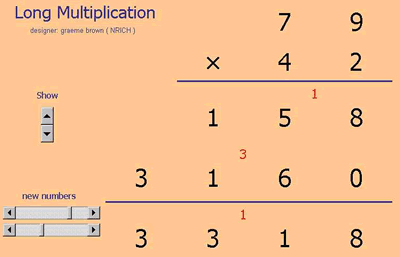# Excel Interactive Resource: Long Multiplication

##### Age 11 to 16Challenge Level

Firstly here's the Excel file: Long Multiplication.xls (Right-click on the link, "Save Target As", and select where you want the file to be saved.)The increment buttons (scroll bars and spinner) change the numbers being multiplied or let you choose whether the result and working shows.

Here's the kind of challenge you might want to try:

First use the Excel file to check your ability to multiply two two-digit numbers.
Make up a few to test yourself.
Perhaps start with easier numbers using only the digits 2, 3, 4, or 5.
Then add a 9 or a 6 somewhere.
How about numbers using the digits 7 or 8?